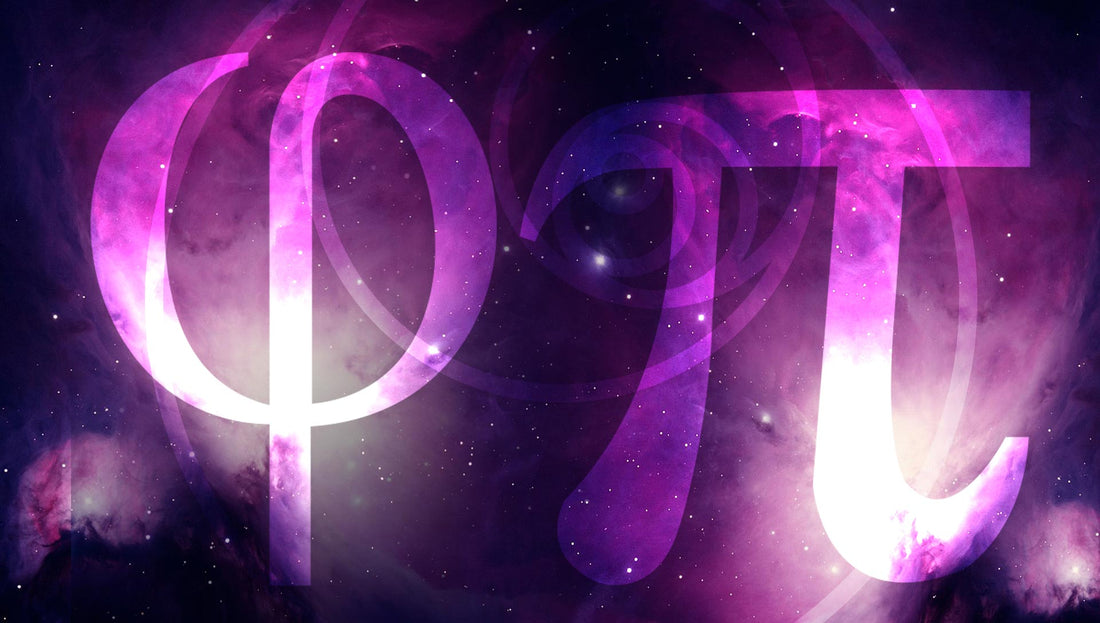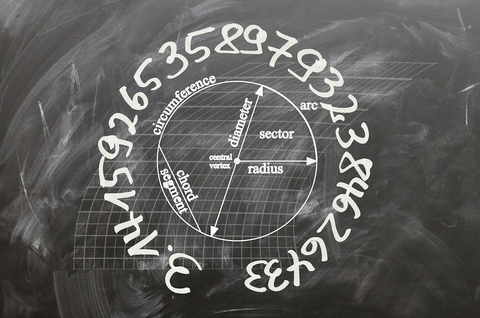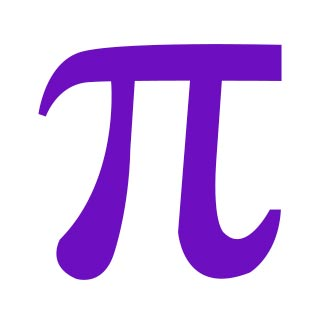# Phi, Pi, and Fibonacci: A Beginner's Guide to The Mathematics of Nature

Nature is incredibly mathematical and finds new ways to demonstrate this to us every day. Although the connection may not jump out at first glance, further investigation reveals the intriguing ways in which mother nature utilizes fractals and ratios to grow symmetrically. Some of these magical ratios and fractals include Phi, Pi, and the Fibonacci Spiral. Understanding how the world around is constructed can aid us in better understanding the underlying meaning and structure behind the cosmos and our surroundings. Let's dive a little further into detail and explore the ratios which compose our physical universe and learn little more about Phi, Pi and the Fibonacci Spiral.

## Phi, Pi, and Fibonacci: A Beginner's Guide to The Mathematics of Nature

Before diving into the intricacies of math in relation to the natural world around us, it's important to understand the basics of these complex concepts.

### What are Fractals?Fractals are complex, infinitely recursive curves or geometric structures in mathematics. A curve or structure that is recursive is one that repeats perfectly through the consistent pattern.

Common fractals include Sierpinski's Triangle, the Dragon Curve, and the Koch Curve. The Fibonacci Spiral is also a fractal, which will be covered further in depth later in this post.

Nature is brimming with fractals. Fractals define the way in which plants grow, such as tree branches, the veins within leaves, the spirals within pinecones. Snowflakes are another example of fractals, as they are perfectly symmetrical on all sides. These complex structures make way for perfection and repetition within nature.

### How are Ratios Relevant?A ratio, structured as 2:1 (for example), is a relation between two numbers which demonstrates how often the second number is contained within the first one. In the example 2:1, we could say there are two flowers for everyone bush.

Although fractals themselves may base their patterns upon any number, fractal dimensions are ratios that depict the complexity of a fractal and the amount of space it takes up as it scales.

Phi (also known as the golden ratio) and Pi are both irrational numbers and mathematical constants. Phi and Pi are more present in our lives than you may realize, which will be covered further in depth later within this post.

## What is the Fibonacci Spiral?The Fibonacci Spiral, also known as the Golden Spiral, is a spiral that gets wider with every quarter turn by a factor of Phi.

In nature, the Fibonacci Spiral is one of the many patterns that presents itself as a fractal. Pinecones, pineapples, sunflowers, lilies, daisies, and roses are perfect examples of fibonacci spirals in nature. The insides of fruits, bananas and apples, also form fibonacci spirals.

Fibonacci Spirals aren't exclusive to plants, of course. Seashells, the markings on animals, the dimensions of the human body, and DNA also benefit from the properties of a Fibonacci Spiral.

Fibonacci Spirals aren't even exclusive to earth, as they are present throughout the solar system, as well.

Phi and Fibonacci Spirals omnipresence throughout life in all knowable forms has merited it's title as the Code of Life, in some circles within the scientific and mathematic community.

## What is Phi?Phi, represented by the Greek character Φ (Phi - pronounced "f-eye"), is an irrational number and mathematical constant with mesmerizing properties. The ratio arises from the proportions of dividing a line into two specific parts so that the whole length of the line divided by it's longer section is equal to the longest section divided by the shortest section.

The equation, a+b/a = a/b roughly equates to 1.618... (or 1.618033988749895...). It is also the solution to the quadratic equation.

Without Phi, the fibonacci spiral it's beautiful patterns throughout nature would not exist. For that reason, it is known as the Golden Ratio or the Divine Ratio, as artists within the renaissance period referred to it as.

## What is Pi?Pi, represented by the Greek character π (Pi - pronounced like "pie"), is an irrational number and mathematical constant originally defined as the ratio between a circle's circumference to it's diameter. The circumference is the distance around the entire circle. The diameter is the length of a line that runs through the center of a circle from one side to it's opposing side.

The equation, circumference/diameter, roughly equates to 3.14... (or 3.14159265358979323846...).

Pi is so important, it is even celebrated as a national holiday on March 14th (3/14).

Although mathematicians have yet to decipher a pattern for the infinite series of digits following the decimal in Pi, it is responsible for many patterns we see within nature.

A zebra's stripes and the spots on a leopard are prime examples of patterns within biology that are defined by Pi, due to their spacing and size according to a study published by The Biophysical Society

There is even a relation between Pi and gravity, according to an article published by WIRED. Through simulations, both the journal of Science and Albert Einstein determined that rivers bend to a ratio of Pi.

While it isn't immediately apparent, nature is incredibly mathematical. Patterns we see every day, the branching of trees, the veins in leaves, the spirals in a pine cone, are all defined by fractals and ratios.

A fractal is a complex and infinitely recursive pattern, and a ratio is a relationship between two numbers that depicts how often the second number is contained within the first.

The Fibonacci Spiral, also known as the Golden Spiral, gets wider with every quarter turn by a factor of the ratio Phi. We see the Fibonacci Spiral all around us without ever even realizing. Pinecones, pineapples, flowers in bloom, and DNA are just a few fibonacci spirals present in nature.

Phi, a+b/a = a/b = 1.618, is also known as the Golden Ratio or Divine Ratio. It is at the very foundation of the Fibonacci Spiral.

Pi, 3.14..., is the ratio between the circumference of a circle and it's diameter. It is such an important mathematical constant, it even has it's own holiday that is recognized every March 14th. Although there appears to be no pattern in it's infinite sequence of digits, it is responsible for many patterns throughout nature.

Fractals and ratios are at the very heart of nature. They allow us to further our understanding of the patterns that surround us not only on our planet, but within our entire solar system.